# Fraction calculator

The calculator performs basic and advanced operations with fractions, expressions with fractions combined with integers, decimals, and mixed numbers. It also shows detailed step-by-step information about the fraction calculation procedure. Solve problems with two, three, or more fractions and numbers in one expression.

## Result:

### 115/8 + 91/2 = 169/8 = 21 1/8 = 21.125

Spelled result in words is one hundred sixty-nine eighths (or twenty-one and one eighth).

### How do you solve fractions step by step?

1. Conversion a mixed number 11 5/8 to a improper fraction: 11 5/8 = 11 5/8 = 11 · 8 + 5/8 = 88 + 5/8 = 93/8

To find new numerator:
a) Multiply the whole number 11 by the denominator 8. Whole number 11 equally 11 * 8/8 = 88/8
b) Add the answer from previous step 88 to the numerator 5. New numerator is 88 + 5 = 93
c) Write a previous answer (new numerator 93) over the denominator 8.

Eleven and five eighths is ninety-three eighths
2. Conversion a mixed number 9 1/2 to a improper fraction: 9 1/2 = 9 1/2 = 9 · 2 + 1/2 = 18 + 1/2 = 19/2

To find new numerator:
a) Multiply the whole number 9 by the denominator 2. Whole number 9 equally 9 * 2/2 = 18/2
b) Add the answer from previous step 18 to the numerator 1. New numerator is 18 + 1 = 19
c) Write a previous answer (new numerator 19) over the denominator 2.

Nine and one half is nineteen halfs
3. Add: 93/8 + 19/2 = 93/8 + 19 · 4/2 · 4 = 93/8 + 76/8 = 93 + 76/8 = 169/8
For adding, subtracting, and comparing fractions, it is suitable to adjust both fractions to a common (equal, identical) denominator. The common denominator you can calculate as the least common multiple of both denominators - LCM(8, 2) = 8. In practice, it is enough to find the common denominator (not necessarily the lowest) by multiplying the denominators: 8 × 2 = 16. In the next intermediate step, the fraction result cannot be further simplified by canceling.
In words - ninety-three eighths plus nineteen halfs = one hundred sixty-nine eighths.

#### Rules for expressions with fractions:

Fractions - use the slash “/” between the numerator and denominator, i.e., for five-hundredths, enter 5/100. If you are using mixed numbers, be sure to leave a single space between the whole and fraction part.
The slash separates the numerator (number above a fraction line) and denominator (number below).

Mixed numerals (mixed fractions or mixed numbers) write as non-zero integer separated by one space and fraction i.e., 1 2/3 (having the same sign). An example of a negative mixed fraction: -5 1/2.
Because slash is both signs for fraction line and division, we recommended use colon (:) as the operator of division fractions i.e., 1/2 : 3.

Decimals (decimal numbers) enter with a decimal point . and they are automatically converted to fractions - i.e. 1.45.

The colon : and slash / is the symbol of division. Can be used to divide mixed numbers 1 2/3 : 4 3/8 or can be used for write complex fractions i.e. 1/2 : 1/3.
An asterisk * or × is the symbol for multiplication.
Plus + is addition, minus sign - is subtraction and ()[] is mathematical parentheses.
The exponentiation/power symbol is ^ - for example: (7/8-4/5)^2 = (7/8-4/5)2

#### Examples:

subtracting fractions: 2/3 - 1/2
multiplying fractions: 7/8 * 3/9
dividing Fractions: 1/2 : 3/4
exponentiation of fraction: 3/5^3
fractional exponents: 16 ^ 1/2
adding fractions and mixed numbers: 8/5 + 6 2/7
dividing integer and fraction: 5 ÷ 1/2
complex fractions: 5/8 : 2 2/3
decimal to fraction: 0.625
Fraction to Decimal: 1/4
Fraction to Percent: 1/8 %
comparing fractions: 1/4 2/3
multiplying a fraction by a whole number: 6 * 3/4
square root of a fraction: sqrt(1/16)
reducing or simplifying the fraction (simplification) - dividing the numerator and denominator of a fraction by the same non-zero number - equivalent fraction: 4/22
expression with brackets: 1/3 * (1/2 - 3 3/8)
compound fraction: 3/4 of 5/7
fractions multiple: 2/3 of 3/5
divide to find the quotient: 3/5 ÷ 2/3

The calculator follows well-known rules for order of operations. The most common mnemonics for remembering this order of operations are:
PEMDAS - Parentheses, Exponents, Multiplication, Division, Addition, Subtraction.
BEDMAS - Brackets, Exponents, Division, Multiplication, Addition, Subtraction
BODMAS - Brackets, Of or Order, Division, Multiplication, Addition, Subtraction.
GEMDAS - Grouping Symbols - brackets (){}, Exponents, Multiplication, Division, Addition, Subtraction.
Be careful, always do multiplication and division before addition and subtraction. Some operators (+ and -) and (* and /) has the same priority and then must evaluate from left to right.

## Fractions in word problems:

• Sum of fractionsWhat is the sum of 2/3+3/5?
• Series and sequencesFind a fraction equivalent to the recurring decimal? 0.435643564356
• Berry SmoothieRory has 5/8 cup of milk. How much milk does she have left after she doubles the recipe of the smoothie? Berry Smoothie: 2 cups strawberries 1 cup blueberries 1/4 cup milk 1 tbsp (tablespoon) sugar 1/2 tsp (teaspoon) lemon juice 1/8 tsp (teaspoon) vanilla
• Rose spendsRose spends 2 1/3 hours studying Math, 1 3/4 hours studying English, and 2 1/4 hours studying Science. Find her average time studying the three subjects.
• Lengths of the poolMiguel swam 6 lengths of the pool. Mat swam 3 times as far as Miguel. Lionel swam 1/3 as far as Miguel. How many lengths did Mat swim?
• Ingrid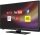Ingrid watched television for 2 1/4 hours, and Devon watched for 3 2/3 hours. For how many hours did they watch television in total?
• A man 3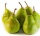A man buys a box of fruits containing 286 fruits out of these 1/2 are apples and the rest are pears. 4/13 of the pears are rotten. He sells the good pears at rupees 4 1/11 each. How much money does he receive on selling the good pears?
• SavingsEva borrowed 1/3 of her savings to her brother, 1/2 of savings spent in the store and 7 euros left. How much did she save?
• Stock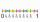Enterprise sold 7/12 of their products to foreign markets and 2/5 of the remainder sold at home. How many % of the products is still in stock?
• Sum three fractionsWork out the sum of 1/4, 1/5 and 3/10.Add two mixed fractions: 2 4/6 + 1 3/6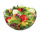We need two tenths kg of a carrot, one tenth of peas and three tenths of of tomatoes to make salad. Express the fraction of the weight of the vegetables to be salad. Convert the result to grams.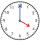Milada took the bus and the journey took 55 minutes. Jarmila was 1h 20 min by train. They arrived in Prague at the same time 10h45 min. At what time did each have to go out?